Disclaimer: This is an example of a student written essay.

Any opinions, findings, conclusions or recommendations expressed in this material are those of the authors and do not necessarily reflect the views of UKEssays.com.

# Cactus Stack Approach with Dijkstra’s Algorithm

 ✅ Paper Type: Free Essay ✅ Subject: Computer Science ✅ Wordcount: 2518 words ✅ Published: 19th Apr 2018

Advance Dijkstra’s Algorithm with Cactus Stack Implementation Logic

Idea Proposed for the Cactus Stack Approach with Dijkstra’s Algorithm

• Palak Kalani
• Nayyar Khan

Abstract— This paper illustrates a possible approach to reduce the complexity and calculation burden that is normally encountered in the Shortest Path Problems. We intend to give a new concept based on the look ahead values of the input nodes given in the shortest path problem as proposed by the famous Djktras Algorithm. A new data structure called as Cactus Stack could be used for the same and we could try to minimize and the loop back issues and traversals in a given graph by looking at the adjacency matrix as well as the creation of a cactus stack which is linked in a listed manner. The calculation of the node values and the total value shall be the same as proposed by the ancient algorithm however by our concept we are trying to reduce the complexity of calculation to a larger extent.

Index Terms—Component, formatting, style, styling, insert. (key words)

## I. Introduction

With the advancement in technology, there is increase in demand for development of industries to fulfill the requirements of the people. But in industries there are a lot of chemicals and lubricants used for equipment some of these chemicals and lubricants are volatile in nature and may cause accidents such as fire. However, fire extinguishers and other preventive measures are also available at the security, but they are not proven executive and we have to flee the area as soon as possible.

This research paper proposes a new algorithm which calculates the shortest path forget away of such problems. The proposed algorithm is based on the original Dijkstra algorithm. This algorithm gives us optimum solution in less time and also reduces the calculation.

## II. Dijkstra Algorithm

Dijkstra was one of the most forceful promoter of programming as a scientific discipline. He has made contribution to the areas of operating systems, programming languages, including deadlock avoidance, contain the notion of structured programming, and algorithms. We will now consider the general problem of finding the length of a shortest path between a and z in an undirected connected simple weighted graph. Dijkstra’s algorithm proceeds by finding the length of a shortest path from a to a first vertex, the length of a shortest path from a to a second vertex, and so on, until the length of a shortest path from a to z is found. As aside benefit, this algorithm is easily extended to find the length of the shortest path from a to all other vertices of the graph, and not just to z.

If you need assistance with writing your essay, our professional essay writing service is here to help!

The algorithm relies on a series of iterations. A distinguished set of vertices is constructed by adding one vertex at each iteration. A labeling procedure is carried out for iteration. In this labeling procedure, a vertex w is labeled with the length of a shortest path from a tow that contains only vertices already in the distinguished set. The vertex added to the distinguished set is one with a minimal label among those vertices not already in the set.

We now give the details of Dijkstra’s algorithm. It begins by labeling a with 0 and the other vertices with ∞. We use the notation L0(a) = 0 and L0(v)=∞for these labels before any iterations have taken place (the subscript 0 stands for the “0th” iteration). These labels are the lengths of shortest paths from a to the vertices, where the paths contain only the vertex a.(Because no path from a to a vertex different from a exists, ∞is the length of a shortest path

1. Between a and this vertex.)Dijkstra’s algorithm proceeds by forming a distinguished set of vertices. Let S k denote this set after k iterations of the labeling procedure. We begin with S0 = ∅. The set Skis formed from Sk−1 by adding a vertex u not in S k−1 with the smallest label.

Lk(a, v) = min{Lk−1(a, v),Lk−1(a, u) + w(u, v)},

ALGORITHM:

Procedure Dijkstra(G: weighted connected simple graph, with

all weights positive)

{G has vertices a = v0, v1, . . . ,vn= z and lengths w(vi , vj )

where w(vi , vj )=∞if {vi , vj} is not an edge in G}

fori := 1 to n

L(vi ) :=∞

L(a) := 0

S :=∅

{the labels are now initialized so that the label of a is 0 and all

other labels are∞, and S is the empty set}

whilezS

u:= a vertex not in S with L(u) minimal

S :=S ∪ {u}

forall vertices v not in S

ifL(u) + w(u, v) < L(v) then L(v) := L(u) + w(u, v)

{this adds a vertex to S with minimal label and updates the

labels of vertices not in S}

returnL(z) {L(z) = length of a shortest path from a to z}

## IV. Limitations of Dijkstra’s Algorithm

Although Dijkstra’s algorithm is an effective algorithm but still there are a lot of circumspections. Some of these are discussed below.

• Presence of calculations bounteously.
• Solutions pleaded by this algorithm are not equitable.
• The reasons for changing paths and beading elements are not favoured.
• It is not explicit when the problem is not closed loop or cyclic i.e. we are having a last element other than destination and last element is connected with only one element then we are not able to reach destination
• It distracts when both next nodes are same then which node we are going to choose for operation.
• We have to check distance or path after one step which is not favourable. For example, if we want to go neemuch from indore and distance of Ujjain from indore is more than distance of devas from indore then according to dijkstra we should go to devas and check distance of desvas between neemuch and if we find more than Ujjain route then we again come back to indore.

## V. Solutions to mentioned limitations

First we use look ahead dijkstra :

## A. Precode

Wnext = min [Wvu ,Wvw]

Look ahead [Wnext ,Wua , Wsub]

{

Wfinal = min[Wnextïƒ Wua, Wnextïƒ Wub]

Return [Wfinal;:a?b]

}

Min[∑_(i=1)^2ã€-(Ti)+Li-1ã€-]

Problem solution Vi=Vj:

Min(P1[∑_(i=1)^2wi], P2[∑_(j=1)^2wj]) =next node

.

## B. Proposed Method

Cs1 pop(a)

{

Cs2(b)

{

Weight (a,b);

}

Cs2(c)

{

Weigth(a,c);

}

Return[min( Cs2(b),Cs2(c))

}

## C. Dijkstra with VFS traversal and cactus stack

VFS(vertical first search)

In the vfs we search in vertical order of cactus stack

f(a,b)≈ f(n,m)

n=a

f(a,’m’)

m=b,c,d,e……..

struct node

{

int*prev;

int*next;

int data;

}

## D. Complexity Comparison

In dijkstra’s complexity is more whereas in proposed method i.e. shortest path with cactus stack is less. In dijkstra’s we need to do calculation from each and every point but in proposed algorithm we need to do calculation from particular points which reduces calculations and complexities.

In mathematics and computer science, an adjacency matrix is a means of representing which vertices (or nodes) of graph are adjacent to which other vertices.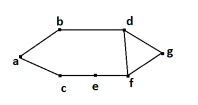Adjacency matrix of above graph is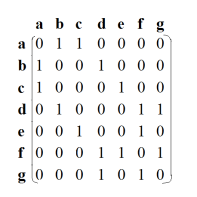The matrix which represents graph with respect to its weight. Now we have to convert matrix into another matrix by using the following program.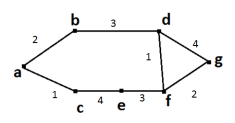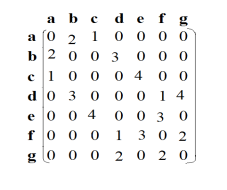CODE :

#include

#include

#define INF 9999

int main( )

{

intarr ;

int cost = {

7, 5, 0, 0,

7, 0, 0, 2,

0, 3, 0, 0,

4, 0, 1, 0

} ;

int i, j, k, n = 4 ;

for ( i = 0 ; i < n ; i++ )

{

for ( j = 0; j < n ; j++ )

{

if ( cost[i][j] == 0 )

arr[i][j] = INF ;

else

arr[i][j] = cost[i][j] ;

}

}

printf ( “Adjacency matrix of cost of edges:n” ) ;

for ( i = 0 ; i < n ; i++ )

{

for ( j = 0; j < n ; j++ )

printf ( “%dt”, arr[i][j] ) ;

printf ( “n” ) ;

}

for ( k = 0 ; k < n ; k++ )

{

for ( i = 0 ; i < n ; i++ )

{

for ( j = 0 ; j < n ; j++ )

{

if ( arr[i][j] >arr[i][k] + arr[k][j] )

arr[i][j] = arr[i][k] + arr[k][j];

}

}

}

Now we take an above example and use this steps to convert into another adjacency matrix: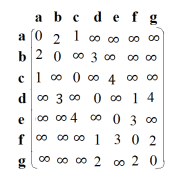.

## G. Traversal of Adjacency Matrix

For solving adjacency matrix, we take the 1st node and observe the row of that node if there is weight on any vertex that means that vertex is connected to 1st node with respective weight.

Similarly we check for all nodes and traverse the matrix.If any vertex have weight infinity that means that vertex is not directly connected to the main vertex whose row is being traverse. If vertex have weight zero that means there is no self loop present.

If user’s last node is not the last node of matrix then we simply traverse the matrix till the node entered by user then we will back traverse rest of node that is we will start traversing last node.

## VI. Cactus Stack

A cactus stack is a set of stacks organized in a systematic format as a tree in which each path from the root to any leaf constitutes a stack.

A Cactus Stack act both like a Tree and a Stack. Like a stack, items can only be added to or removed from only one end that is top of the cactus stack; like a Tree, nodes in the Cactus Stack may have parent child relationships. Cactus Stacks are traversed from the child nodes to the parent nodes rather than vice-versa, as in a Binary Search Tree. One of the strongest benefits of a cactus stack is that it allows parallel data structures to exist with the same root.

## A. Creation of Cactus Stack

Let us understand this matrix with the help of an example showed above. Series of steps should be as following:

First, We should know the starting and ending point. Let us assume that a is the starting point and f is the ending point. Then we put vertices of graph in cactus stack. Put a in cs1. Now a is connected to b and c. so b and c are put in cs2. b is connected to d and c is connected to e so we put d and e in cs3. Repeat same step till the last node is traversed. On traversing the adjacency matrix if two adjacent node has weight other than infinity and zero then we put that nodes in different cactus stacks.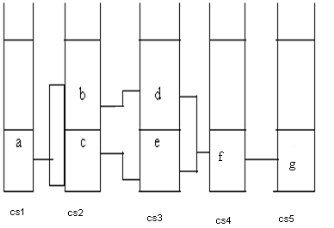## B. Linkage in Cactus Stack

After plotting the vertices in cactus stacks. If there is connection between element of cs1, cs2 then we have to join them. Similarly we will join elements of each stack to its consecutive stack.

## VII. Conclusion

With the help of Cactus Stack and Linked List for the shortest path the time complexity is reduced theoretically than the theory proposed by Dijkshtra’s Shortest path algorithm. Thus we conclude that time complexity is reduced.

## References

List and number all bibliographical references in 9-point Times, single-spaced, at the end of your paper. When referenced in the text, enclose the citation number in square brackets, for example: . Where appropriate, include the name(s) of editors of referenced books. The template will number citations consecutively within brackets . The sentence punctuation follows the bracket . Refer simply to the reference number, as in “”—do not use “Ref. ” or “reference ”. Do not use reference citations as nouns of a sentence (e.g., not: “as the writer explains in ”).

Our academic experts are ready and waiting to assist with any writing project you may have. From simple essay plans, through to full dissertations, you can guarantee we have a service perfectly matched to your needs.

Unless there are six authors or more give all authors’ names and do not use “et al.”. Papers that have not been published, even if they have been submitted for publication, should be cited as “unpublished” . Papers that have been accepted for publication should be cited as “in press” . Capitalize only the first word in a paper title, except for proper nouns and element symbols.

For papers published in translation journals, please give the English citation first, followed by the original foreign-language citation .

1. Wang Tian-yu, The Application of the Shortest Path Algorithm in the Evacuation System, 2011 International Conference of Information Technology, Computer Engineering and Management Sciences (references)
2. Kenneth H. Rosen, Discrete_Mathematics_and_Its_Applications_7th_Edition_Rosen, page-710-713
3. Fuhao Zhang, Improve On Dijkshtra’s Shortest Path Algorithm for Huge Data
4. R. Nicole, “Title of paper with only first word capitalized,” J. Name Stand. Abbrev., in press.
5. Y. Yorozu, M. Hirano, K. Oka, and Y. Tagawa, “Electron spectroscopy studies on magneto-optical media and plastic substrate interface,” IEEE Transl. J. Magn. Japan, vol. 2, pp. 740–741, August 1987 [Digests 9th Annual Conf. Magnetics Japan, p. 301, 1982].
6. IEEE JOURNAL OF SOLID-STATE CIRCUITS, VOL. 41, NO. 8, AUGUST 2006 1803 ”Phase Noise and Jitter in CMOS Ring Oscillators”, Asad A. Abidi. pp1803-1816.
7. M. Young, The Technical Writer’s Handbook. Mill Valley, CA: University Science, 1989.

View all

## DMCA / Removal Request

If you are the original writer of this essay and no longer wish to have your work published on UKEssays.com then please:

Related Services

Prices from

OMR57

Approximate costs for:

• 1000 words
• 7 day deliveryHumanity University

Dedicated to your worth and value as a human being!

Related Lectures